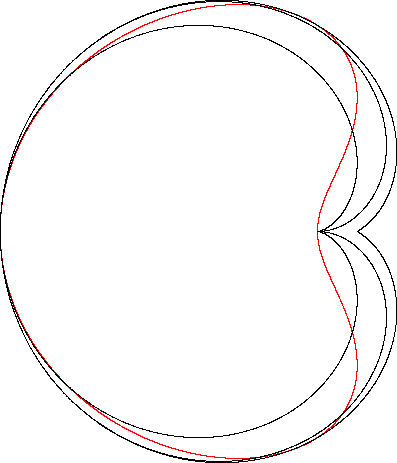Lima Bean Curve
LIMA BEAN CURVE

Balmoral Software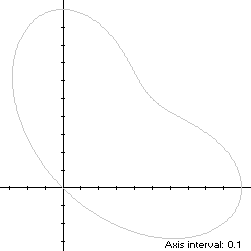The lima bean curve is a tilted closed curve with polar equation
r(t) = sin3(t) + cos3(t), 0 ≤ t < π
The curve is traced around and through the origin in a counterclockwise direction, starting from its maximum abscissa point (1,0) at t = 0, reaching its closest approach to the origin in the first quadrant at (1/2,1/2) when t = π/4, then its maximum ordinate point (0,1) at t = π/2 and through Quadrant II to the origin at t = 3π/4 before returning through Quadrant IV to its starting point. Its minimum abscissa and ordinate points are (-0.282887,0.621170) and (0.621170,-0.282887), respectively, so its bounding rectangle is a 1.282887 x 1.282887 square.

### Metrics

The perimeter of the lima bean curve is 3.931703 and its area is 5π/16. Its centroid is the point (3/10,3/10).

### Rotated Curve

Since
r(π/4 + t) = r(π/4 - t),
the lima bean curve is symmetric with respect to the line y = x, and is more amenable to analysis after being rotated clockwise by π/4, producing an x-symmetric curve S:The polar function of the rotated curve is:
rS(t) = sin3(t + π/4) + cos3(t + π/4), 0 ≤ t < π,
and the corresponding coordinate functions are
x(t) = rS(t)cos(t) = [sin3(t + π/4) + cos3(t + π/4)]cos(t)

y(t) = rS(t)sin(t) = [sin3(t + π/4) + cos3(t + π/4)]sin(t)

The minimum abscissa point of S is the origin at t = π/2; and its two maximum abscissa points areat t = π/6 and t = 5π/6, so S and the lima bean curve are non-convex by the multiple local extrema test. The maximum ordinate point of S is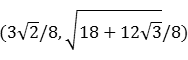The bounding rectangle for inconics is delimited by the ordinate extrema and the cusp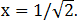### Convex Hull

The convex hull is created by connecting the maximum abscissa points with a vertical line segment of length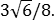We haveso by (L2), the perimeter of the convex hull is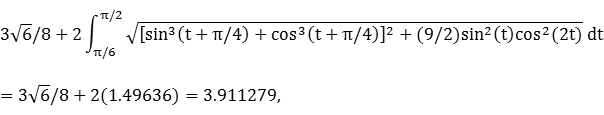which is less than 1% shorter than that of the lima bean curve.

The line segment of the convex hull creates an isosceles triangle with the origin, having area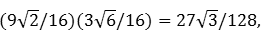as shown in blue in the diagram above. The centroid abscissa of this triangle is the average of its vertex abscissas, or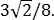By (A2), the remainder of the convex hull has area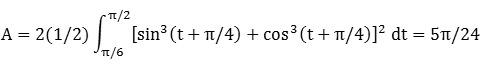By (C2), the associated centroid abscissa is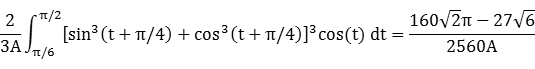The convex hull component metrics can be summarized as follows:
 Area Centroid abscissa Product Region Triangle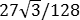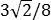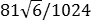Remainder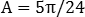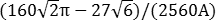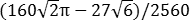Total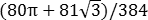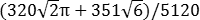The area of the convex hull is 1.019853, about 4% larger than that of the lima bean curve.

The centroid abscissa of the convex hull of S is the weighted average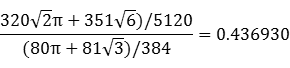After a counterclockwise rotation by π/4, the centroid's position with respect to the lima bean curve is (0.308956,0.308956).

### Incircle

A candidate incircle is located on the x-axis between the origin and the cusp, and so has radius R and center abscissa c both equal to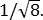For verification, we have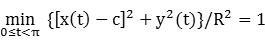After a counterclockwise rotation by π/4, the incircle's center is (1/4,1/4).

### Inellipse

Using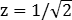in Lemma E,
d/dt [x(t) - z]y(t) = d/dt (-1/8)sin3(t)[-2cos(t) - 3cos(3t) + cos(5t)]
has a zero at t* = 1.245619. The corresponding coordinates are
x* = 0.201781

y* = 0.598499

We then have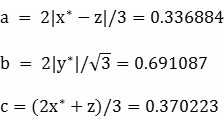For verification,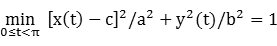### Circumellipse

Using z = 0 in Lemma E,
d/dt [x(t) - z]y(t) = d/dt [26sin(2t) + 2sin(4t) - 6sin(6t) + sin(8t)]/64
has a zero at t* = π/4. The corresponding coordinates are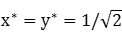We then haveFor verification,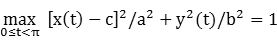### Circumcircle

The radius of a circle centered on the x-axis and circumscribing S is at least its maximum ordinate, so a candidate for the circumcircle has radius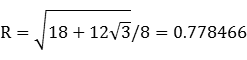and center abscissa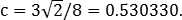For verification,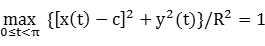After a counterclockwise rotation by π/4, the circumcircle's center is (3/8,3/8).

### Summary Table

 Perimeter Area Centroid Figure Parameters Incircle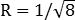2.221442 0.392699 (0.25,0.25) Inellipse * a = 0.336884b = 0.691087 3.326054 0.731414 (0.370223,0) Lima bean curve Width: 1.282887Height: 1.282887 3.931703 0.981748 (0.3,0.3) Convex hull 3.911279 1.019853 (0.308956,0.308956) Circumellipse *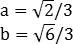4.119019 1.209201 (0.471405,0) Circumcircle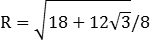4.891247 1.903835 (0.375,0.375)

*: With respect to rotated curve S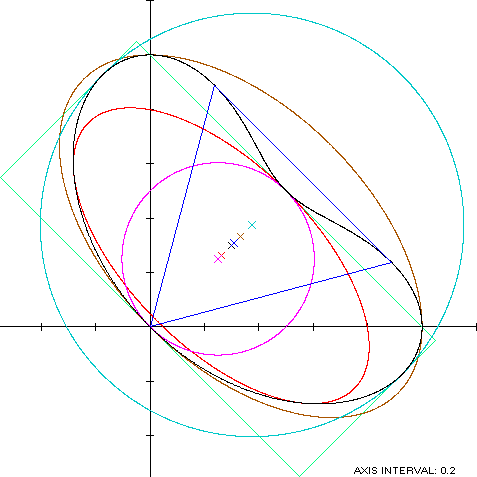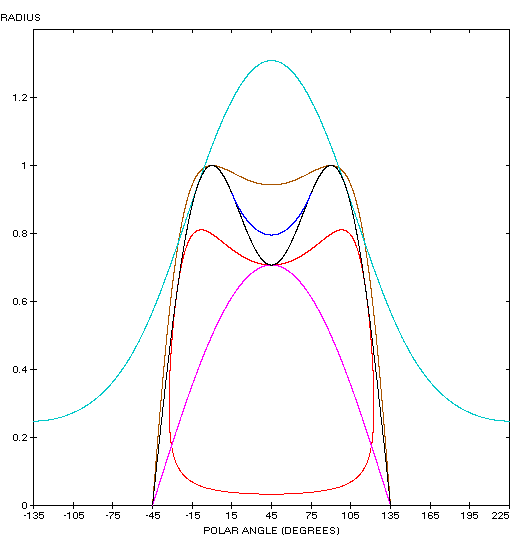The rotated lima bean curve (red) is a member of a group of similarly-shaped figures described on these pages, including (inside to outside) the cardioid, the cochleoid and the Cayley sextic.